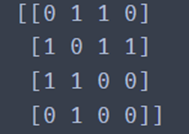﻿ 关于networkx返回图的邻接矩阵问题_python_脚本之家
python# 关于networkx返回图的邻接矩阵问题

## networkx返回图的邻接矩阵

### 这里只举个小例子

```import networkx as nx  # 导入必要的函数包
import scipy as sp
import operator
import matplotlib.pyplot as plp

h = nx.Graph()  # 我这里需要用到的图
h.add_edges_from([(1, 2), (1, 3), (1, 5), (1, 4), (2, 8), (2, 6), (3, 6), (4, 7)])

nx.draw_networkx(h)  # draw
plp.show()

```ok，一个简简单单的邻接矩阵解决完毕。

## NetworkX学习笔记之邻接矩阵

### 邻接矩阵

```As = nx.adjacency_matrix(G)
print(As)
``````# 转化成二维数组形式的矩阵
A = As.todense()
print(A)
```### 已知图的邻接矩阵，创建图

```import numpy as np
A = np.array([[0, 1, 1], [1, 0, 1], [1, 1, 0]])
G = nx.from_numpy_matrix(A)
nx.draw(G, node_size=800, with_labels=True)
```